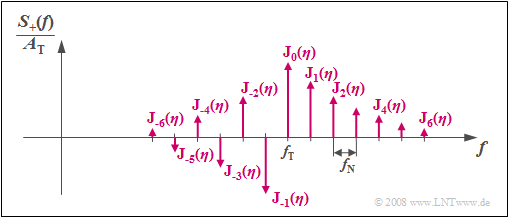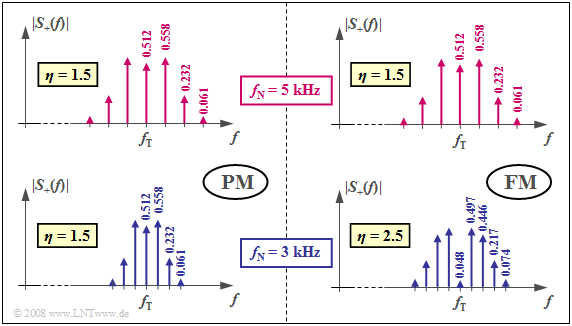# Bessel Functions of the First Kind

## Applet Description

This applet allows the calculation and graphical representation of the Bessel functions of the first kind and  $n$–th order according to the series representation:

$${\rm J}_n (x) = \sum\limits_{k=0}^{\infty}\frac{(-1)^k \cdot (x/2)^{n \hspace{0.05cm} + \hspace{0.05cm} 2 \hspace{0.05cm}\cdot \hspace{0.05cm}k}}{k! \cdot (n+k)!} \hspace{0.05cm}.$$
• The functions  ${\rm J}_n (x)$  can be represented graphically for the order  $n=0$  to  $n=9$  in different colors.
• The left output provides the function values  ${\rm J}_0 (x = x_1)$, ... , ${\rm J}_9 (x = x_1)$  for a slider-settable value  $x_1$  in the range  $0 \le x_1 \le 15$  with increment  $0.5$.
• The right output provides the function values  ${\rm J}_0 (x = x_2)$, ... , ${\rm J}_9 (x = x_2)$  for a slider-settable value  $x_2$  (same range and value increment like on the left).

## Theoretical Background

### General Information about the Bessel Functions

Bessel functions (or cylinder functions) are solutions of the Bessel differential equation of the form

$$x^2 \cdot \frac{ {\rm d}^2}{{\rm d}x^2}\ {\rm J}_n (x) \ + \ x \cdot \frac{ {\rm d}}{{\rm d}x}\ {\rm J}_n (x) \ + \ (x^2 - n^2) \cdot {\rm J}_n (x)= 0.$$

This is an ordinary second-order linear differential equation.  The parameter  $n$  is usually integer, also in this program.  These mathematical functions, introduced by  Friedrich Wilhelm Bessel  in 1844, can also be represented in closed form as integrals:

$${\rm J}_n (x) = \frac{1}{2\pi}\cdot \int_{-\pi}^{+\pi} {{\rm e}^{\hspace{0.05cm}{\rm j}\hspace{0.05cm}\cdot \hspace{0.05cm}[\hspace{0.05cm}x \hspace{0.05cm}\cdot \hspace{0.05cm}\sin(\alpha) -\hspace{0.05cm} n \hspace{0.05cm}\cdot \hspace{0.05cm}\alpha \hspace{0.05cm}]}}\hspace{0.1cm}{\rm d}\alpha \hspace{0.05cm}.$$

The functions  ${\rm J}_n (x)$  belong to the class of Bessel functions of the first kind.  The parameter  $n$  is called the "order".

Note:   There are a number of modifications of the Bessel functions, including the Bessel functions of the second kind named  ${\rm Y}_n (x)$.  For integer  $n$,  ${\rm Y}_n (x)$  can be replaced by  ${\rm J}_n (x)$  functions.  However, in this applet, only the first kind Bessel functions  ${\rm J}_n (x)$  are considered.

### Properties of the Bessel Functions

$\text{Property (A):}$   If the function values for  $n = 0$  and  $n = 1$  are known, then the Bessel function for  $n ≥ 2$  can be determined iteratively:

$${\rm J}_n (x) ={2 \cdot (n-1)}/{x} \cdot {\rm J}_{n-1} (x) - {\rm J}_{n-2} (x) \hspace{0.05cm}.$$

$\text{Example (A):}$   Let  ${\rm J}_0 (x = 2) = 0.22389$  and  ${\rm J}_1 (x= 2) = 0.57672$.  From this it can be calculated iteratively:

$${\rm J}_2 (x= 2) ={2 \cdot 1}/{2} \cdot {\rm J}_{1} (x= 2) - {\rm J}_{0} (x= 2) = 0.57672 - 0.22389 = \hspace{0.15cm}\underline{0.35283}\hspace{0.05cm},$$
$${\rm J}_3 (x= 2) ={2 \cdot 2}/{2} \cdot {\rm J}_{2} (x= 2) - {\rm J}_{1} (x= 2) = 2 \cdot 0.35283 - 0.57672 = \hspace{0.15cm}\underline{0.12894}\hspace{0.05cm},$$
$${\rm J}_4 (x= 2) ={2 \cdot 3}/{2} \cdot {\rm J}_{3} (x= 2) - {\rm J}_{2} (x= 2) = 3 \cdot 0.12894 - 0.35283 = \hspace{0.15cm}\underline{0.03400}\hspace{0.05cm}.$$

$\text{Property (B):}$   The symmetry relationship applies  ${\rm J}_{–n}(x) = (–1)^n · {\rm J}_n(x)$:

$${\rm J}_{-1}(x) = - {\rm J}_{1}(x), \hspace{0.3cm}{\rm J}_{-2}(x) = {\rm J}_{2}(x), \hspace{0.3cm}{\rm J}_{-3}(x) = - {\rm J}_{3}(x).$$

$\text{Example (B):}$   For the spectrum of the analytic signal, the following applies to the case  "Phase Modulation of a sinusoidal signal":Phase Modulation:   Spectrum of the Analytic Signal
$$S_{\rm +}(f) = A_{\rm T} \cdot \sum_{n = - \infty}^{+\infty}{\rm J}_n (\eta) \cdot \delta \big[f - (f_{\rm T}+ n \cdot f_{\rm N})\big]\hspace{0.05cm}.$$

Denote this

• $f_{\rm T}$  the carrier frequency   (German:   $\rm T$rägerfrequenz),
• $f_{\rm N}$  the frequency of the source signal   (German:   $\rm N$achrichtenfrequenz),
• $A_{\rm T}$  the carrier amplitude   (German:   $\rm T$rägeramplitude).

Parameter of the Bessel functions in this application is the modulation index  $\eta$.

Based on the graphic, the following statements are possible:

• $S_+(f)$  consists here of an infinite number of discrete lines at a distance of $f_{\rm N}$.
• Thus the sectrum is in principle infinitely extended.
• The weights of the spectral lines at $f_{\rm T} + n · f_{\rm N}$  $(n$ integer$)$  are determined by the modulation index  $η$  over the Bessel functions  ${\rm J}_n(η)$.
• The spectral lines are real in the case of a sinusoidal source signal and a cosinusoidal carrier and symmetrical about $f_{\rm T}$  for even  $n$.
• In the case of odd  $n$, a change of sign corresponding to  $\text{Property (B)}$  must be taken into account.
• The phase modulation of another phase oscillation of source and / or carrier signal provides the same magnitude spectrum.

### Applications of the Bessel Functions

The applications of the Bessel functions in nature and engineering are diverse and play an important role in physics, for example:

• Electromagnetic waves in a cylindrical waveguide,
• Solutions to the radial Schroedinger equation,
• Pressure amplitudes of inviscid rotational flows,
• Heat conduction in a cylindrical object,
• Diffusion problems on a lattice,
• Dynamics of floating bodies,
• Frequency-dependent friction in circular pipelines,
• Angular resolution.

The Bessel functions belong to the "Special Functions" because of their many applications in mathematical physics.

In the following, we restrict ourselves to a few areas that are addressed in our tutorial  $\rm LNTwww$.

$\text{Example (C):} \hspace{0.5cm} \text{Use in Spectral Analysis} \ \Rightarrow \ \text{Kaiser-Bessel filter}$

The  Spectral Leakage Effect  is the falsification of the spectrum of a periodic and thereby time unlimited signal due to the implicit time limitation of the discrete Fourier transform (DFT).  This will be done, for example, by a spectrum analyzer

• in the time signal not existing frequency components simulated, and/or
• actually existing spectral components are obscured by sidelobes.

The task of the  Spectral Analysis  is to limit the influence of the spectral leakage effect by providing suitable window functions.

Such a window function provides, for example, the Kaiser–Bessel window   ⇒   see section  Special Window Functions.  Its time-discrete window function with the Bessel function zero order   ⇒   ${\rm J}_0(x)$, the parameter  $\alpha=3.5$  and the window length  $N$:

$$w_\nu = \frac{ {\rm J}_0\big(\pi \cdot \alpha \cdot \sqrt{1 - (2\nu/N)^2}\big)}{ {\rm J}_0\big(\pi \cdot \alpha \big)}.$$

On the page  Quality Criteria of Window Functions  the parameters of the Kaiser-Bessel window are given.

• The large "Minimum Distance between the main lobe and side lobes" and the desired small "maximum Scaling Error" are favourable.
• Due to the very large "Equivalent Noise Width" the Kaiser-Bessel window cuts in the most important comparison criterion "Maximum Process Loss" but worse than the established Hamming and Hanning windows.

$\text{Example (D):} \hspace{0.5cm} \text{Rice Fading Channel Model}$

The  Rayleigh distribution  describes the mobile channel on the assumption that there is no direct path and thus the multiplicative factor  $z(t) = x(t) + {\rm j} \cdot y(t)$  is composed solely of diffusely scattered components.

In the case of a direct component  (Line of Sight, LoS) one has to add in the model DC components  $x_0$  and/or  $y_0$  for the mean-free Gaussian processes  $x(t)$  and  $y(t)$ :

$$x(t) \hspace{0.1cm} \Rightarrow \hspace{0.1cm} x(t) +x_0 \hspace{0.05cm},$$
$$y(t) \hspace{0.1cm} \Rightarrow \hspace{0.1cm} y(t) +y_0\hspace{0.05cm},$$
$$z(t) = x(t) + {\rm j} \cdot y(t)\hspace{0.1cm}\Rightarrow \hspace{0.1cm} z(t) +z_0 \hspace{0.05cm},$$
$$z_0 = x_0 + {\rm j} \cdot y_0\hspace{0.05cm}.$$

The graph shows the Rice fading channel model.  It can be summarized as follows:

• The real part  $x(t)$  is Gaussian with mean  $x_0$  and variance  $\sigma ^2$.
• The imaginary part  $y(t)$  is also Gaussian  $($mean  $y_0$,  equal variance  $\sigma ^2)$  and independent of  $x(t)$.
• For  $z_0 \ne 0$, the magnitude  $\vert z(t)\vert$  is Rice distributed, from which the term "Rice Fading" arises.
• To simplify the notation, we set  $\vert z(t)\vert = a(t)$.  For $a < 0$ , the pdf is  $f_a(a) \equiv 0$, for  $a \ge 0$  the following equation holds, where  ${\rm I_0}(x)$  is the modified Bessel function of zero order:
$$f_a(a) = \frac{a}{\sigma^2} \cdot {\rm e}^{ - (a^2 + \vert z_0 \vert ^2)/(2\sigma^2)} \cdot {\rm I}_0 \left [ \frac{a \cdot \vert z_0 \vert}{\sigma^2} \right ] \hspace{0.5cm}\text{with}\hspace{0.5cm}{\rm I }_0 (u) = {\rm J }_0 ({\rm j} \cdot u) = \sum_{k = 0}^{\infty} \frac{ (u/2)^{2k} }{k! \cdot \Gamma (k+1)} \hspace{0.05cm}.$$
• Between the modified Bessel function  ${\rm J_0}(x)$  and the traditional Bessel function  ${\rm I_0}(x)$  of first kind the following relation exists:  ${\rm I }_0 (u) = {\rm J }_0 ({\rm j} \cdot u)$.

$\text{Example (E):} \hspace{0.5cm} \text{Analysis of the Frequency Spectrum of Frequency Modulated Signals}$

$\text{Example (B)}$  has already been shown that the angle modulation of a harmonic oscillation of the frequency $f_{\rm N}$  leads to a line spectrum.  The spectral lines are around the carrier frequency $f_{\rm T}$  at  $f_{\rm T} + n \cdot f_{\rm N}$  with  $n \in \{ \ \text{...}, -2, -1, \ 0, +1, +2, \text{...} \ \}$.  The weights of the Dirac lines are  ${\rm J }_n(\eta)$, depending on the modulation index  $\eta$.

The graph shows the magnitude spectrum  $\vert S_{\rm +}(f) \vert$  of the analytic signal in phase modulation (PM) and frequency modulation (FM), two different forms of angle modulation (WM).  Bessel lines with values less than  $0.03$  are neglected in both cases.Discrete spectra with phase modulation (left) and frequency modulation (right)

For the upper half of the graphic, the modulator parameters are chosen so that for each $f_{\rm N} = 5 \ \rm kHz$  here is a Bessel spectrum with the modulation index  $η = 1.5$.  Disregarding the phase relationships, the same spectra and the same signals result for both systems.

The lower graphics apply with otherwise identical settings for the same message frequency $f_{\rm N} = 3 \ \rm kHz$.  One notices:

• In phase modulation, the spectral function is narrower than  $f_{\rm N} = 5 \ \rm kHz$, because the distance of the Bessel lines is now only  $3 \ \rm kHz$.  Since the PN modulation index is independent of $f_{\rm N}$, the same bessel weights result as in $f_{\rm N} = 5 \ \rm kHz$.
• Also in the frequency modulation, the Bessel lines now occur at a distance of  $3 \ \rm kHz$.  However, since FM has a modulation index inversely proportional to $f_{\rm N}$, there are now significantly more Bessel lines at the bottom due to the larger modulation index  $η = 2.5$ than in the upper right chart  $($for  $η = 1.5$  valid$)$.

## Applet Manual

(A)     Sum formula of the Bessel functions  ${\rm J}_n(x)$

(B)     Selection of the order  $n$  for the graphical representation

(C)     Plot area of the Bessel functions

(D)     Variation of the graphic representation

$\hspace{1.5cm}$"$+$" (Enlarge),

$\hspace{1.5cm}$ "$-$" (Decrease)

$\hspace{1.5cm}$ "$\rm o$" (Reset to default)

$\hspace{1.5cm}$ "$\leftarrow$" (pushed to the left), usw.

(E)     Selection of the abscissa value  $x_1$  for the left numeric output

(F)     Numerical output of the Bessel function values  ${\rm J}_n(x_1)$

(G)     Selection of the abscissa value  $x_2$ for  the right numeric output

(F)     Numerical output of the Bessel function values  ${\rm J}_n(x_2)$

## About the Authors

This interactive applet was designed and realized at the  Institute for Communications Engineering  at the  Technical University of Munich.